Courses

# Test: Moment Of A Force - 1

## 25 Questions MCQ Test Engineering Mechanics - Notes, Videos, MCQs & PPTs | Test: Moment Of A Force - 1

Description
This mock test of Test: Moment Of A Force - 1 for Mechanical Engineering helps you for every Mechanical Engineering entrance exam. This contains 25 Multiple Choice Questions for Mechanical Engineering Test: Moment Of A Force - 1 (mcq) to study with solutions a complete question bank. The solved questions answers in this Test: Moment Of A Force - 1 quiz give you a good mix of easy questions and tough questions. Mechanical Engineering students definitely take this Test: Moment Of A Force - 1 exercise for a better result in the exam. You can find other Test: Moment Of A Force - 1 extra questions, long questions & short questions for Mechanical Engineering on EduRev as well by searching above.
QUESTION: 1

### Determine the smallest force applied at R which creates the same moment about P as by 75N.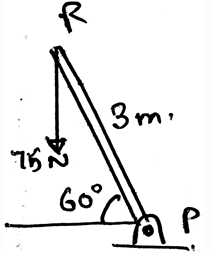Solution:

As we know that the moment is the cross product of the distance and the force we will try to apply the same here. We see that the perpendicular distance is 3m. Thus we get the distance. And hence multiply it with the force, the moment = 112.5Nm. Because the force component perpendicular to the distance need to be taken.

QUESTION: 2

### What does the moment of the force measure?

Solution:

The moment of the force measures the tendency of the rotation of the body along any axis, whether it be the centroid axis of the body, or any of the outside axis. The couple moment is produced by two forces, not by a single force. The total work done is the dot product of force and distance not the cross.

QUESTION: 3

### If a car is moving forward, what is the direction of the moment of the moment caused by the rotation of the tires?

Solution:

When you curl your wrist in the direction in which the tires are moving then you will find that the thumb is pointing outwards. That is outwards the body of the car. This phenomena is also observed in rainy seasons. When cars travel on the roads, the water is thrown outside from the tires, due to moment.

QUESTION: 4

The tendency of rotation of the body along any axis is also called ___________

Solution:

The tendency of rotation of the body along any axis also called the torque. It is the moment of the force acting perpendicular to the direction of the axis of rotation. If the axis and the force are meeting at any point then there is no moment applied by the force.

QUESTION: 5

The moment of the force is the product of the force and the perpendicular distance of the axis and the point of action of the force.

Solution:

The moment is the product of the force applied to the body and the perpendicular distance of the point of action of the force to the axis about which the body is being rotated. That is the moment is the cross product of the force and the distance between the axis and the point of action.

QUESTION: 6

Determine the moment about the point T.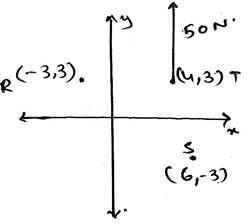Solution:

As we know that the moment is the cross product of the distance and the force we will try to apply the same here. We see that the perpendicular distance is 0m. Thus we get the distance. And hence multiply it with the force, 0x50.

QUESTION: 7

The moment axis is in the direction perpendicular to the plane of the force and the distance.

Solution:

The moment axis is always perpendicular to the planes of the force and the distance of the axis and the point of action of the force on the body. This means that the moment is the cross product of the force and the distance between the axis and the point of action of the force.

QUESTION: 8

Find the moment along T.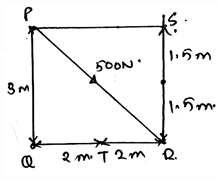Solution:

As we know that the moment is the cross product of the distance and the force we will try to apply the same here. But here we have number of forces components acting on the T point. So by adding all the moments caused by all the forces will give us the value as 600Nm.

QUESTION: 9

If you are getting to know about the direction of the moment caused by the force applied on the body by using your wrist and curling it in the direction of the rotation then which of the following is not right?

Solution:

The curled hand represents various thing. The direction of the moment axis is given by the thumb. The direction of the force is given by the fingers. As we place the fingers on the force and curl towards the rotational direction of the body about the axis.

QUESTION: 10

The moment axis, force and the perpendicular distance in the moment of the force calculation is lying in____________

Solution:

The moment axis, force and the perpendicular distance is lying in the three dimensional Cartesian. It doesn’t lye on the single plane. It also doesn’t lye in a single line. Nor in the direction of the force. Thus they all lye in the planes which are perpendicular to each other.

QUESTION: 11

If the rotation is clockwise in this page, suppose, then in which direction will the thumb project if you curl your hand in the same direction of the rotation?

Solution:

As the curling will give the direction perpendicular to the paper. But it does depend upon the rotation sense. In this example, the sense is clockwise. Thus the thumb goes into the paper. That is it goes away from the viewer. Thus the answer.

QUESTION: 12

Which of the following is true?

Solution:

The moment is the vector quantity. Thus the value of the total moment caused by various forces acting on the body is the vector sum of all the vectors. Also the moments are not perpendicular to each other, unless it is specified. Thus assumptions cant be taken for the direction of the moment.

QUESTION: 13

Determine the moment about the point R.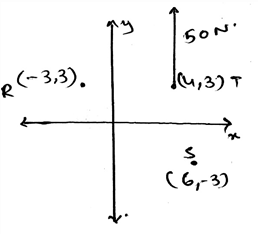Solution:

As we know that the moment is the cross product of the distance and the force we will try to apply the same here. We see that the perpendicular distance is 7m. Thus we get the distance. And hence multiply it with the force, 7×50

= 350 Nm.

QUESTION: 14

Find the moment along Q.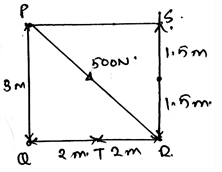Solution:

As we know that the moment is the cross product of the distance and the force we will try to apply the same here. But here we have number of forces components acting on the T point. So by adding all the moments caused by all the forces will give us the value as 1200Nm.

QUESTION: 15

Determine the moment of the force along point P.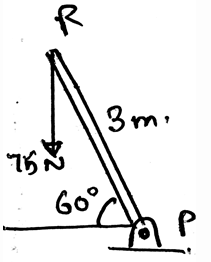Solution:

As we know that the moment is the cross product of the distance and the force we will try to apply the same here. We see that the perpendicular distance is 1.5 (3cos60). Thus we get the distance. And hence multiply it with the force, 75×1.5. Because the force component perpendicular to the distance need to be taken.

QUESTION: 16

Find the moment of the force about the point R.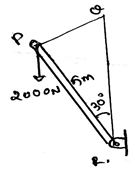Solution:

As we know that the moment is the cross product of the distance and the force we will try to apply the same here. We see that the perpendicular distance is 5m. Thus we get the distance. And hence multiply it with the force, 5x2000xsin30. Because the force component perpendicular to the distance need to be taken.

QUESTION: 17

How far from the shaft at P a 200N vertical force must act so as to create the same moment as produced by the 75N, at P?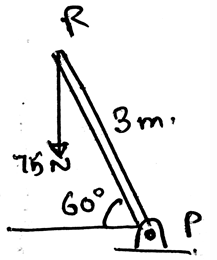Solution:

As we know that the moment is the cross product of the distance and the force we will try to apply the same here. So let the distance be y, then the force of 200N will act downward. The distance perpendicular is xcos60. Thus equating the 112.5 to 200ycos60.

QUESTION: 18

Find the moment along the point at the mid of the line SR.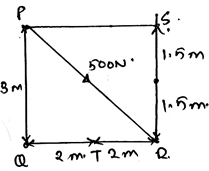Solution:

As we know that the moment is the cross product of the distance and the force we will try to apply the same here. But here we have number of forces components acting on the T point. So by adding all the moments caused by all the forces will give us the value as 600Nm.

QUESTION: 19

Which of the following is true?

Solution:

We can take such assumptions. We are free to do so. We just need to specify if clockwise is positive then the counter clockwise is to be negative. Also such assumption are very useful in 2D calculation of the moments caused by various forces on the body.

QUESTION: 20

The moment is the cross product of which two vectors?

Solution:

The cross product needs to take in the proper sequence. If not taken then the answer is just the opposite of the true answer. That’s why, the answer is not the Force and Radius vectors, but the Radius and Force vectors. Because the moment has its direction, as many of the cross products have, and thus precaution needs to be taken.

QUESTION: 21

The ___________ forces do not cause the rotation.

Solution:

The concurrent forces are the which are somewhere touching the axis of rotation. If any of the force is touching that axis, that force is not considered, or is insufficient to cause a rotation. If a force is concurrent then the perpendicular distance of the force from the line of axis is zero, thus no rotation. As we know rotation is caused by moment.

QUESTION: 22

Choose the correct one.

Solution:

The coplanar system means the consideration is with the 2D only. No 3D. Thus if there is any moment needed to be considered we need to take it along the point and not along the axis. But talking about the axis, there might be no axis in the 2D, as it is only in the 3D.

QUESTION: 23

Determine the moment about the point S.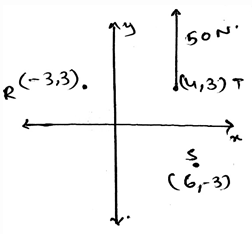Solution:
QUESTION: 24

Moments can be added like scalars that is it can be added algebraically with proper signs.

Solution:

The moments in the 2D can be added algebraically just like the scalar quantities. But the need is that the signs must be taken into the consideration. As if clockwise is positive, so anti clockwise is negative. And the result can be negative too. That is counter clockwise direction.

QUESTION: 25

If you are opening the door, then the force you apply is greater if you open it from the part closer to the hinges.

Solution:

As the moment is the product of the force and the perpendicular distance of the point of action and the axis, thus more the distance more will be the moment. And hence to overcome that you have to apply more energy. Thus the answer.